100,360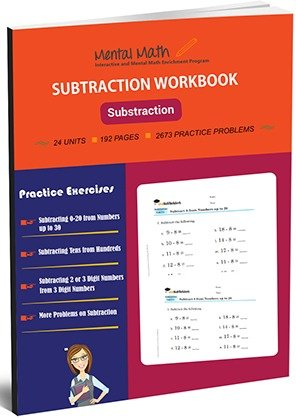\$24.00 \$14.00

\$10 off if you buy whole book!

# The Most Effective Subtraction Worksheets on the Market

In Mental Math’s subtraction worksheets, your child will quickly and easily learn subtracting 0 to 20, subtracting tens from hundreds and more other problems on subtraction.

Choose from a wide variety of innovative worksheets that best fit your child’s level and needs. World problems help your child solve daily life problems and further develop their math skills.

Created by leading math experts, our progressive worksheets include 192 pages, 2673 practice problems and 24 units.

Our progressive worksheets ensure that your child will quickly master each math concept, and develop the math proficiency needed to move to the next level.

#### Features of our worksheets ......................................................

• Completely covers the appropriate math curriculum of each Level.
• Helps children achieve higher math skills in a step-by-step and gradual way at their own pace.
• 10-20 page worksheets with sufficient math problems that avoids repetition.
• Contains many samples for allowing children to learn better problem solving.
• Encourages children to build self-learning habits.
• Includes challenging math problems.
• And much, much more!

### Now, you can purchase the most affordable and effective math worksheets developed by the leading math curriculum experts in the country in just \$1 for 10-27 pages

Worksheet Topics/Curriculum # of Pages Add to cart
Unit 1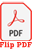Subtracting with 1 8 pages
Unit-2Subtracting with 2 8 pages
Unit-3Subtracting with 3 8 pages
Unit-4Subtracting with 4 8 pages
Unit-5Subtracting with 5 8 pages
Unit-6Subtracting with 6 and 7 8 pages
Unit-7Subtracting with 8 and 9 8 pages
Unit-8Subtracting 0-9 Randomly 8 pages
Unit-9Subtracting 0-10 from Numbers up to 30 8 pages
Unit-10Subtracting 0-12 from Numbers up to 30 8 pages
Unit-11Subtracting 0-14 from Numbers up to 30 8 pages
Unit-12Subtracting 0-16 from Numbers up to 30 8 pages
Unit-13Subtracting 0-17 from Numbers up to 30 8 pages
Unit-14Subtracting 0-18 from Numbers up to 30 8 pages
Unit-15Subtracting 0-19 from Numbers up to 30 8 pages
Unit-16Subtracting 0-20 from Numbers up to 30 8 pages
Unit-17Subtracting 1 Digit Numbers in Vertical Form 8 pages
Unit-18Subtracting 1 or 2 Digit Numbers from 2 Digit Numbers 8 pages
Unit-19Subtracting 2 Digit Numbers from 2 Digit Numbers 8 pages
Unit-20Subtracting Tens from Hundreds 8 pages
Unit-21Subtracting 3 Digit Numbers from 3 Digit Number 8 pages
Unit-22Subtracting 2 or 3 Digit Numbers from 3 Digit Numbers 8 pages
Unit-23Subtracting Numbers Ending in 0 or with a 0 in the Middle 8 pages
Unit-24More Problems on Subtraction 8 pages

## Choose From Over 8 Worksheets That Best Suit Your Child’s Skill Level and Unique Needs

You can purchase our high quality math worksheets separately, or purchase an entire book and save 40%!

#### Equation Worksheets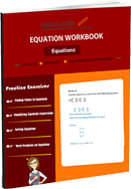Evaluate Algebraic Expressions and Solve Equations

See all units

#### Integers WorksheetsFour Operations on integers and their Simplification

See all units

#### Decimals Worksheets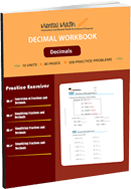Decimal Concept and Four Operations on Decimals

See all units

#### Fractions Worksheets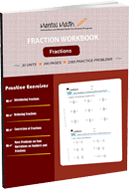Fraction Concepts and Four Operations on Fractions

See all units

#### Division Worksheets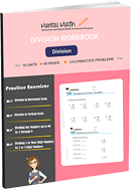Dividing up to 4 or more digit Numbers by 2 or 3-digit Numbers

See all units

#### Multiplication Worksheets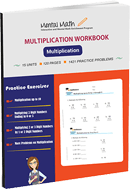Multiplication Table of 2 through 10 and Multiplication by Thousands

See all units

#### Subtraction Worksheets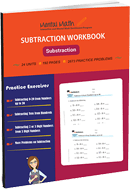Subtracting 4 to 1-digit Numbers form 4 digit Numbers

See all units

## Need More Convincing? Read What a Few Grateful Parents Have to Say:###### Alison R.